Get instant live expert help with Excel or Google Sheets“My Excelchat expert helped me in less than 20 minutes, saving me what would have been 5 hours of work!”

#### Post your problem and you’ll get expert help in seconds.

Your message must be at least 40 characters
Our professional experts are available now. Your privacy is guaranteed.

# How to Lookup a Value Between Two Numbers in Excel

Excel allows a user to lookup a value between two numbers using the LOOKUP function. This step by step tutorial will assist all levels of Excel users in learning how to lookup a value between two numbers in Excel.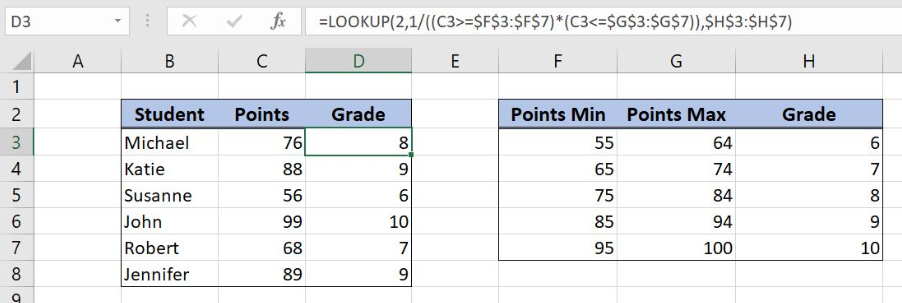Figure 1. The result of the nested LOOKUP function

## Syntax of the LOOKUP Formula

The generic formula for the LOOKUP function is:

`=LOOKUP(lookup_value, lookup_vector, [result_vector])`

The parameters of the LOOKUP function are:

• lookup_value – a value or column based on which we want to get a value
• lookup_vector – an array of vectors for getting the values
• [result_vector] – an array of resulting values.

## Setting up Our Data for the LOOKUP Function

The first table consists of 3 columns: “Student” (column B), “Ponts” (column C) and “Grade” (column D). The second table is the grade scale and has 3 columns: “Points Min” (column F), “Points Max” (column G) and “Grade” (column F). Based on a number of the points, we want to get a grade for each student in column D, using the points scale in the second table.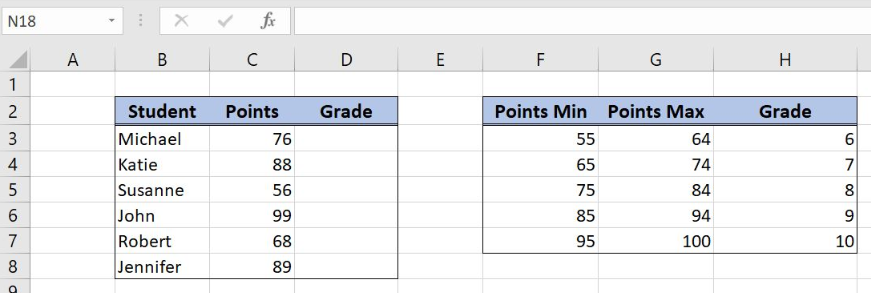Figure 2. The tables for the LOOKUP function example

## Get a Grade Based on Points Using the LOOKUP Function

In our example, we want to get a grade in the cell D3 for Michael, who has 76 points. In order to do this, we need to check the second table and to find where the 76 points are between “Points Min” and “Points Max”.

The formula looks like:

`=LOOKUP(2, 1/((C3>=\$F\$3:\$F\$7) * (C3<=\$G\$3:\$G\$7)), \$H\$3:\$H\$9)`

The parameter lookup_value is 2. The lookup_vector is `1/((C3>=\$F\$3:\$F\$7) * (C3<=\$G\$3:\$G\$7))`. The result vector is \$H\$3:\$H\$9.

To apply the LOOKUP function, we need to follow these steps:

• Select cell D3 and click on it
• Insert the formula: `=LOOKUP(2, 1/((C3>=\$F\$3:\$F\$7) * (C3<=\$G\$3:\$G\$7)), \$H\$3:\$H\$9)`
• Press enter
• Drag the formula down to the other cells in the column by clicking and dragging the little “+” icon at the bottom-right of the cell.

Let’s evaluate the formula and see what happens: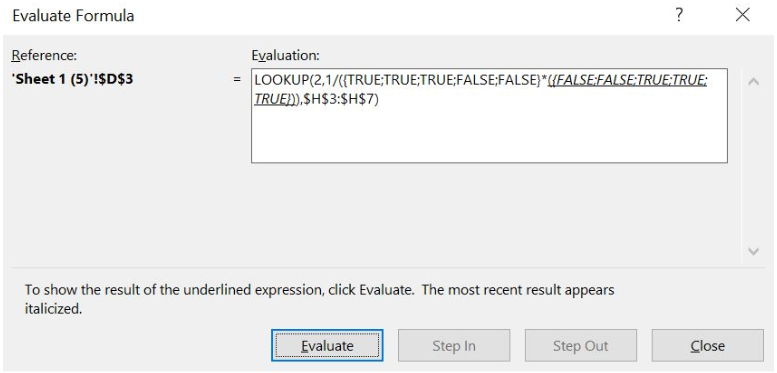Figure 3. Evaluate the formula – step 1

First, values from the lookup_value are compared with ranges F3:F7 and G3:G7 and TRUE or FALSE are returned for both comparations.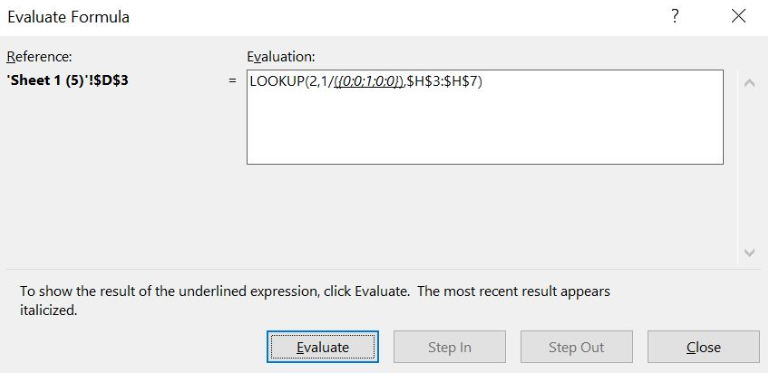Figure 4. Evaluate the formula – step 2

Next, all TRUE values are converted to 1 and FALSE to 0. Now, the two arrays are multiplied and we get the array of 0 and 1. Next, we have to divide 1 with this array.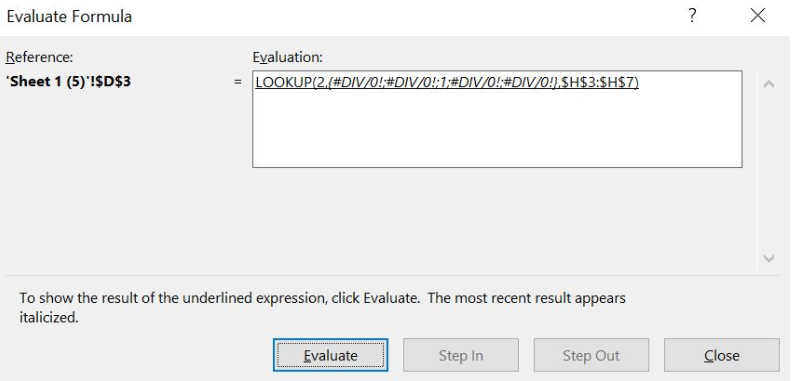Figure 5. Evaluate the formula – step 3

After the division, we get an array of division by zero errors and one 1. The position of that 1, is the row of our result_vector where the resulting value is.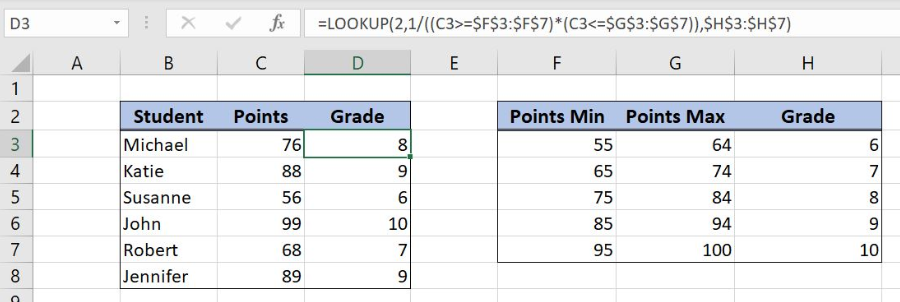Figure 6. Using the LOOKUP function to get a value between two numbers

As you can see in Figure 6, the value of 76 points is between 75 and 84 (F5 and G5). Therefore, we get the grade from the cell H5, which is 8.

Most of the time, the problem you will need to solve will be more complex than a simple application of a formula or function. If you want to save hours of research and frustration, try our live Excelchat service! Our Excel Experts are available 24/7 to answer any Excel question you may have. We guarantee a connection within 30 seconds and a customized solution within 20 minutes.

Solution examplesUse the Vlookup Function to complete the "employee" column of table 2. Use "job Id" from table 2 as your lookup_value(s) and table 1 as your reference.
Solved by C. H. in 16 minsIf a cell in another sheet is populated I need a vlookup done. If the cell is not populated I need the cell to return blank.
Solved by T. D. in 60 minsI am trying to make a chart that turns a week range red if nothing is entered in the range. If something is entered then I would like it to turn green. Please Help
Solved by E. U. in 43 minsI need a check box to show/hide an answer of an if function
Solved by Z. U. in 23 minsI need a formula to compare the data in two columns and then export the mismatched data in the 3rd column
Solved by S. Q. in 20 mins